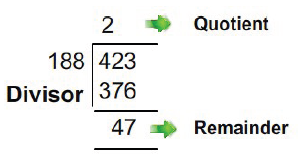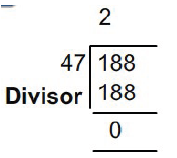# O Level Exam

#### GCD (Greatest Common Division) of two numbers

Problem Definition: To find the Greatest Common Divisor (GCD)of two numbers.

Problem Analysis: GCD of two numbers is the greatest common factor of the given two numbers.

GCD of two numbers is obtained using the following method: Divide the larger of the two numbers by the smaller one.

Divide the divisor by the remainder.

Repeat this process till the remainder becomes zero.

The last divisor is the GCD of the two.

Solving by Example: Consider two numbers 188 and 423.

The smaller number is 188.Therefore divide 423 by 188.

Step 147(Remainder) becomes the divisor and 188 (Divisor) becomes the dividend.

Step 2Since the remainder has become zero, 47 is the GCD of 188 and 423.

Algorithm Definition

Step 1: Start

Step 2: Read two numbers for which GCD is to be found, say (a,b).

Step 3: Let a be the larger of the two numbers.

Step 4: Divide a by b.

Step 5: Get the remainder of the division operation.

Step 6: Divide the divisor of the previous division operation with the remainder.

Step 7: Repeat steps 4,5,6 till the remainder become zero.

Step 8: The divisor of the last division operation performed is the GCD of the two numbers.

Step 9: Stop